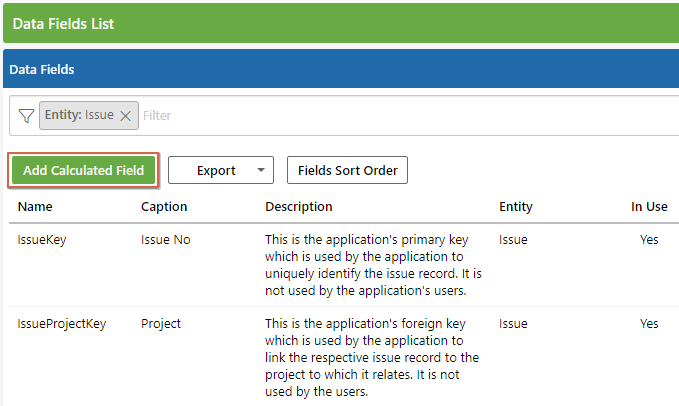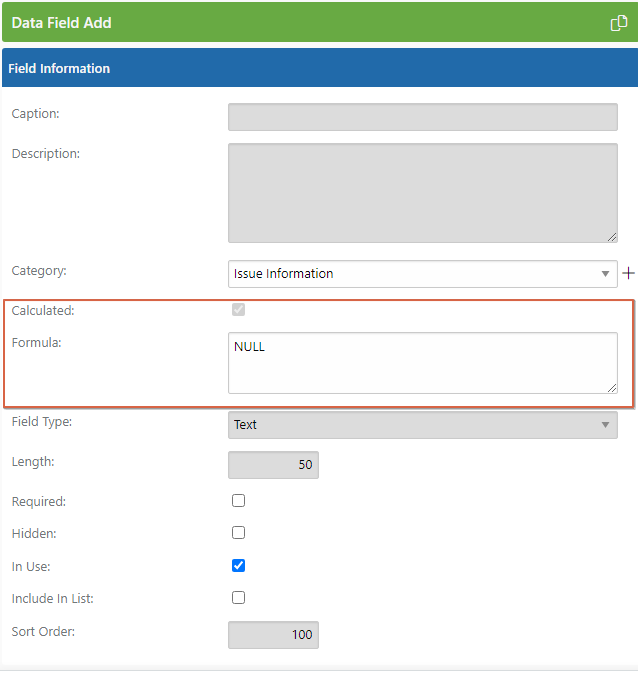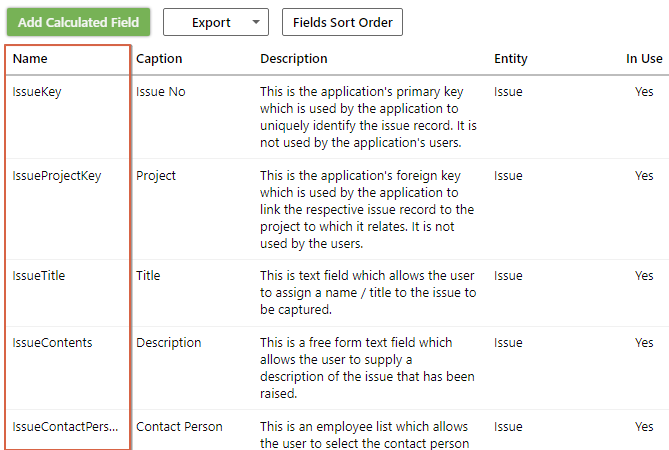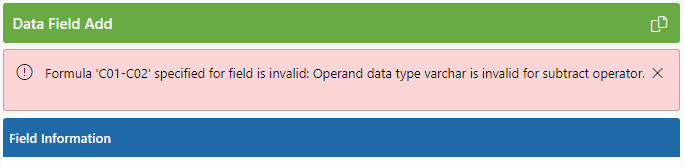Follow

# Calculated Fields

This article provides some background information on the Calculated Fields available in PPO:

Calculated fields are a powerful feature within PPO that allow you to calculate the value of a field on the basis of a formula. Although this feature can be very useful, it should be used with care.

Some examples of possible calculated fields include:

• Net present value (NPV);
• Gross profit % per project;
• Balance of budget available;
• Automatic prioritisation ranking;
• Schedule Performance Indicator (SPI);
• Cost Performance Indicator (CPI);
• Return on investment (ROI); or
• Number of days an issue has been open

• Select the applicable entity;
• Click on the "Add Calculated Field" button;Configuring a calculated field is done in the same way that you would configure any other custom field (for further details refer to the following knowledge base article).

You will however notice that there is an additional setting for Formula as shown in the screenshot above.The formula that you provide should use standard SQL syntax and should reference the underlying field names as they exist in the PPO database. It, therefore, does require some knowledge of the database structure as well as of SQL.

If you have a decent knowledge of SQL functions you can write the easier formulas yourself. Complex calculations that require assistance from the Support Team are available and can be requested by logging a support ticket from your PPO Home Page.

The following tips and guidelines may be of assistance when creating your own formulas:

• You can determine the actual field name for a field (as opposed to its caption) by referring to the PPO Field Name on the Data Fields List. It is the PPO Field Name that needs to be used in formulas.• All field names in PPO are unique, so you generally do not have to worry about ambiguous field names when tables are joined. The only exceptions are the special fields called Created (the last time the item was updated) and UserKey (the user that updated the item) which are common across all tables. If you want to use these fields in a formula you need to prefix it with the table name (the letters tbl and the entity name) e.g. tblIssue.Created.
• The maximum length of the formula is 255 characters. If you need more complicated functionality, it is recommended that you create a user-defined function on the database and call that instead.
• If there is a possibility that the value of a field that you reference in your formula could be NULL it is recommended that you use the COALESCE or ISNULL functions to provide a default value.
• Although the validity of the formula is checked against the table at the time that it is created and subsequent field changes are checked to ensure that it does not impact on the validity of a formula, care should be taken when using formulas.
• Ensure that the field type that you choose for the calculated field is compatible with the result generated by your formula.
• Be careful of division by zero errors. Always check whether the divisor is zero.
• When referring to custom fields in a formula you should explicitly cast or convert them to the correct type since they are defined as varchar fields in the database.
• The underlying data values for RAG indicators are as follows: 0=Grey1=Red2=Amber3=Green.
• You can refer to other calculated fields by putting the field name in curly braces e.g. CostVariance is calculated so if you want to calculate the variance as a percentage of budget, you can use the formula CASE WHEN CostBudget = 0 THEN 0 ELSE {CostVariance} / CostBudget END

A lot of examples and further detail are provided in the following knowledge base article.

### Limitations

Any valid SQL can be used, except literal strings, comments and command delimiters.  These limitations are there to protect the database from SQL injection attacks.

If there is a problem with your formula PPO will prevent you from saving the calculated data field and will report an error in the formula.### Risks

PPO allows any valid SQL in a calculated field and although PPO tries its best to ensure that formula's do not break the PPO instance, we cannot check for all eventualities.  Some of the things that you should keep in mind are:

• There is a performance impact, especially when you are referencing other tables or doing aggregates. On large instances, this impact could be considerable. If you experience slow page loads, complex calculated fields are usually to blame.  Contact the PPO Support Desk to assist in optimising the calculation or adding appropriate indexes.
• If you reference other fields in a calculation and you then take that field out of use or change its field type, the calculation is likely to break.  Therefore, if you have many calculated fields, consider the impact on calculated fields before making any changes. PPO provides functionality to help you understand where a field is used.
• Complex calculated fields are difficult for users to understand. Make sure that you put a good description in the field details so that users can understand how the values were derived.

### General Guidelines

The following general guidelines may be useful:

• Fields may contain NULL values, especially in the case of custom fields. It is usually a good idea to explicitly convert NULL values to 0 when using the field in a calculation by using the syntax ISNULL(P01,0);
• When working with custom fields, it is generally necessary to convert the field to a type that is compatible with the field type used in PPO. For example in the case of a currency field in PPO, you should convert the field to a money field e.g. CONVERT(money,C01). When working with decimals, you should use CONVERT(decimal(17,2),P01). For integers, you can use CONVERT(int,P01)
• When calculating percentages or doing any division, you should check that the denominator is not 0 (or NULL). You can do this with a CASE statement e.g. CASE WHEN C02 = 0 THEN 0 ELSE C01/C02 * 100 END. When incorporating the rules above, the full statement would be CASE WHEN ISNULL(C02,0) = CONVERT(money,0) THEN 0 ELSE CONVERT(money,ISNULL(C01,0))  / CONVERT(money,ISNULL(C02,0)) * 100 END
• When referring to other tables within a calculation, it is usually prudent to fully qualify field names, especially when referring to fields that are used on all tables like MasterKey and Created. e.g. CONVERT(money,ISNULL((SELECT SUM(c.CostBudget) FROM tblCost c WHERE c.CostProjectKey = tblProjects.ProjectKey AND c.MasterKey IS NULL),0))

### Legacy calculated fields

In May 2017 we introduced a change that allows you to add an unlimited number of calculated fields as described above.  Before this change, you had to use one of the available custom fields to implement a custom calculated field.

If you are currently still using custom fields for calculations and you want to free up some of these fields to use as non-calculated fields, they can be migrated to the new "virtual" calculated fields.

If you want to migrate your current calculated fields, simply log a support call and the support team will gladly assist you.